Open Access. Powered by Scholars. Published by Universities.®

# Applied Mathematics Commons™

Open Access. Powered by Scholars. Published by Universities.®

5,980 Full-Text Articles 6,863 Authors 1,902,317 Downloads224 Institutions

## All Articles in Applied Mathematics

5,980 full-text articles. Page 201 of 205.

Projektowanie I Optymalizacja Procesów Proj., 2010Wroclaw University of Technology

#### Projektowanie I Optymalizacja Procesów Proj., Wojciech M. Budzianowski

##### Wojciech Budzianowski

No abstract provided.

Metody Numeryczne Lab., 2010Consulting Services

#### Metody Numeryczne Lab., Wojciech M. Budzianowski

##### Wojciech Budzianowski

No abstract provided.

Odnawialne Źródła Energii W., 2010Wroclaw University of Technology

#### Odnawialne Źródła Energii W., Wojciech M. Budzianowski

##### Wojciech Budzianowski

No abstract provided.

Numerical Simulations Of Two-Dimensional Fractional Subdiffusion Problems, 2010Hong Kong Baptist University

#### Numerical Simulations Of Two-Dimensional Fractional Subdiffusion Problems, Hermann Brunner, L. Ling, Masahiro Yamamoto

##### Department of Mathematics Journal Articles

The growing number of applications of fractional derivatives in various fields of science and engineering indicates that there is a significant demand for better mathematical algorithms for models with real objects and processes. Currently, most algorithms are designed for 1D problems due to the memory effect in fractional derivatives. In this work, the 2D fractional subdiffusion problems are solved by an algorithm that couples an adaptive time stepping and adaptive spatial basis selection approach. The proposed algorithm is also used to simulate a subdiffusion-convection equation

Parallelization Of The Wolff Single-Cluster Algorithm, 2010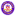University of Latvia

#### Parallelization Of The Wolff Single-Cluster Algorithm, Jevgenijs Kaupužs, Jānis Rimšāns, Roderick V.N. Melnik

##### Mathematics Faculty Publications

A parallel [open multiprocessing (OpenMP)] implementation of the Wolff single-cluster algorithm has been developed and tested for the three-dimensional (3D) Ising model. The developed procedure is generalizable to other lattice spin models and its effectiveness depends on the specific application at hand. The applicability of the developed methodology is discussed in the context of the applications, where a sophisticated shuffling scheme is used to generate pseudorandom numbers of high quality, and an iterative method is applied to find the critical temperature of the 3D Ising model with a great accuracy. For the lattice with linear size L=1024, we have ...

Comparison Study Of Dynamics In One-Sided And Two-Sided Solid-Combustion Models, 2010Bridgewater State University

#### Comparison Study Of Dynamics In One-Sided And Two-Sided Solid-Combustion Models, Y. Yang, Laura K. Gross, J. Yu

##### Mathematics and Computer Science Faculty Publications

Comparing two-sided and one-sided solid-combustion models, this paper concerns nonlinear transition behavior of small disturbances of front propagation and temperature as they evolve in time. Features include linear instability of basic solutions and weakly nonlinear evolution of small perturbations, as well as the complex dynamics of period doubling, quadrupling, and eventual chaotic oscillations. Both asymptotic and numerical methods are used for different solution regimes. First, multiscale weakly nonlinear analysis takes into account the cumulative effect of small nonlinearities to obtain a correct description of the evolution over long times. For a range of parameters, the asymptotic method with some dominant ...

Fast Computation Of Kernel Estimators, 2010University of Pennsylvania

#### Fast Computation Of Kernel Estimators, Vikas C. Raykar, Ramani Duraiswami, Linda H. Zhao

##### Statistics Papers

The computational complexity of evaluating the kernel density estimate (or its derivatives) at m evaluation points given n sample points scales quadratically as O(nm)—making it prohibitively expensive for large datasets. While approximate methods like binning could speed up the computation, they lack a precise control over the accuracy of the approximation. There is no straightforward way of choosing the binning parameters a priori in order to achieve a desired approximation error. We propose a novel computationally efficient ε-exact approximation algorithm for the univariate Gaussian kernel-based density derivative estimation that reduces the computational complexity from O(nm) to ...

Periodic Solutions Of Neutral Delay Integral Equations Of Advanced Type, 2010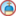University of Dayton

#### Periodic Solutions Of Neutral Delay Integral Equations Of Advanced Type, Muhammad Islam, Nasrin Sultana, James Booth

##### Mathematics Faculty Publications

We study the existence of continuous periodic solutions of a neutral delay integral equation of advanced type. In the analysis we employ three fixed point theorems: Banach, Krasnosel'skii, and Krasnosel'skii-Schaefer. Krasnosel'skii-Schaefer fixed point theorem requires an a priori bound on all solutions. We employ a Liapunov type method to obtain such bound.

Coarsening In High Order, Discrete, Ill-Posed Diffusion Equations, 2010University of Dayton

#### Coarsening In High Order, Discrete, Ill-Posed Diffusion Equations, Catherine Kublik

##### Mathematics Faculty Publications

We study the discrete version of a family of ill-posed, nonlinear diffusion equations of order 2n. The fourth order (n=2) version of these equations constitutes our main motivation, as it appears prominently in image processing and computer vision literature. It was proposed by You and Kaveh as a model for denoising images while maintaining sharp object boundaries (edges). The second order equation (n=1) corresponds to another famous model from image processing, namely Perona and Malik's anisotropic diffusion, and was studied in earlier papers. The equations studied in this paper are high order analogues of the Perona-Malik equation ...

2010University of Dayton

#### Linearly Ordered Topological Spaces And Weak Domain Representability, Joe Mashburn

##### Mathematics Faculty Publications

It is well known that domain representable spaces, that is topological spaces that are homeomorphic to the space of maximal elements of some domain, must be Baire. In this paper it is shown that every linearly ordered topological space (LOTS) is homeomorphic to an open dense subset of a weak domain representable space. This means that weak domain representable spaces need not be Baire.

Skyrmions, Rational Maps & Scaling Identities, 2010Aristotle University of Thessaloniki

#### Skyrmions, Rational Maps & Scaling Identities, E. G. Charalampidis, T. A. Ioannidou, N. S. Manton

##### Mathematics and Statistics Department Faculty Publication Series

Starting from approximate Skyrmion solutions obtained using the rational map ansatz, improved approximate Skyrmions are constructed using scaling arguments. Although the energy improvement is small, the change of shape clarifies whether the true Skyrmions are more oblate or prolate.

Oscillatory And Monotonic Modes Of Long-Wave Marangoni Convection In A Thin Film, 2010Western Kentucky University

#### Oscillatory And Monotonic Modes Of Long-Wave Marangoni Convection In A Thin Film, Sergey Shklyaev, Mikhail Khenner, Alexei Alabuzhev

##### Mikhail Khenner

We study long-wave Marangoni convection in a layer heated from below. Using the scaling k=OBi, where k is the wave number and Bi is the Biot number, we derive a set of amplitude equations. Analysis of this set shows presence of monotonic and oscillatory modes of instability. Oscillatory mode has not been previously found for such direction of heating. Studies of weakly nonlinear dynamics demonstrate that stable steady and oscillatory patterns can be found near the stability threshold.

2010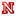University of Nebraska - Lincoln

#### From Energy Gradient And Natural Selection To Biodiversity And Stability Of Ecosystems, Bo Deng

##### Faculty Publications, Department of Mathematics

The purpose of this paper is to incorporate well-established ecological principles into a foodweb model consisting of four trophic levels --- abiotic resources, plants, herbivores, and carnivores. The underlining principles include Kimura's neutral theory of genetic evolution, Liebig's Law of the Minimum for plant growth, Holling's functionals for herbivore foraging and carnivore predation, the One-Life Rule for all organisms, and Lotka-Volterra's model for intraand interspecific competitions. Numerical simulations of the model led to the following statistical findings: (a) particular foodwebs can give contradicting observations on biodiversity and productivity, in particular, all known functional forms -- - positive, negative, sigmoidal ...

Bass’ Nk Groups And Cd H-Fibrant Hochschild Homology, 2010Ciudad Universitaria Pab 1

#### Bass’ Nk Groups And Cd H-Fibrant Hochschild Homology, G. Cortiñas, C. Haesemeyer, Mark E. Walker, C. Weibel

##### Faculty Publications, Department of Mathematics

The K-theory of a polynomial ring R[t ] contains the K-theory of R as a summand. For R commutative and containing Q, we describe K(R[t ])/K(R) in terms of Hochschild homology and the cohomology of Kähler differentials for the cdh topology.

We use this to address Bass’ question, whether Kn(R) = Kn(R[t ]) implies Kn(R) = Kn(R[t1, t2]). The answer to this question is affirmative when R is essentially of finite type over the complex numbers, but negative in general.

Traveling Wave Solutions For A Nonlocal Reaction-Diffusion Model Of Influenza A Drift, 2010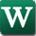Wright State University - Main Campus

#### Traveling Wave Solutions For A Nonlocal Reaction-Diffusion Model Of Influenza A Drift, Joaquin Riviera, Yi Li

##### Mathematics and Statistics Faculty Publications

In this paper we discuss the existence of traveling wave solutions for a nonlocal reaction-diffusion model of Influenza A proposed in Lin et. al. (2003). The proof for the existence of the traveling wave takes advantage of the different time scales between the evolution of the disease and the progress of the disease in the population. Under this framework we are able to use the techniques from geometric singular perturbation theory to prove the existence of the traveling wave.

Multigrid In A Weighted Space Arising From Axisymmetric Electromagnetics, 2010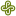Portland State University

#### Multigrid In A Weighted Space Arising From Axisymmetric Electromagnetics, Dylan M. Copeland, Jay Gopalakrishnan, Minah Oh

##### Mathematics and Statistics Faculty Publications and Presentations

Consider the space of two-dimensional vector functions whose components and curl are square integrable with respect to the degenerate weight given by the radial variable. This space arises naturally when modeling electromagnetic problems under axial symmetry and performing a dimension reduction via cylindrical coordinates. We prove that if the original three-dimensional domain is convex then the multigrid Vcycle applied to the inner product in this space converges, provided certain modern smoothers are used. For the convergence analysis, we first prove several intermediate results, e.g., the approximation properties of a commuting projector in weighted norms, and a superconvergence estimate for ...

Hybridization And Postprocessing Techniques For Mixed Eigenfunctions, 2010University of Minnesota - Twin Cities

#### Hybridization And Postprocessing Techniques For Mixed Eigenfunctions, Bernardo Cockburn, Jay Gopalakrishnan, F. Li, Ngoc Cuong Nguyen, Jaume Peraire

##### Mathematics and Statistics Faculty Publications and Presentations

We introduce hybridization and postprocessing techniques for the Raviart–Thomas approximation of second-order elliptic eigenvalue problems. Hybridization reduces the Raviart–Thomas approximation to a condensed eigenproblem. The condensed eigenproblem is nonlinear, but smaller than the original mixed approximation. We derive multiple iterative algorithms for solving the condensed eigenproblem and examine their interrelationships and convergence rates. An element-by-element postprocessing technique to improve accuracy of computed eigenfunctions is also presented. We prove that a projection of the error in the eigenspace approximation by the mixed method (of any order) superconverges and that the postprocessed eigenfunction approximations converge faster for smooth eigenfunctions. Numerical ...

A Class Of Discontinuous Petrov–Galerkin Methods. Part I: The Transport Equation, 2010University of Texas at Austin

#### A Class Of Discontinuous Petrov–Galerkin Methods. Part I: The Transport Equation, Leszek Demkowicz, Jay Gopalakrishnan

##### Mathematics and Statistics Faculty Publications and Presentations

Considering a simple model transport problem, we present a new finite element method. While the new method fits in the class of discontinuous Galerkin (DG) methods, it differs from standard DG and streamline diffusion methods, in that it uses a space of discontinuous trial functions tailored for stability. The new method, unlike the older approaches, yields optimal estimates for the primal variable in both the element size h and polynomial degree p, and outperforms the standard upwind DG method.

On The Accuracy Of Explicit Finite-Volume Schemes For Fluctuating Hydrodynamics, 2010Lawrence Livermore National Laboratory

#### On The Accuracy Of Explicit Finite-Volume Schemes For Fluctuating Hydrodynamics, Aleksandar Donev, Eric Vanden-Eijnden, Alejandro Garcia, John B. Bell

##### Alejandro Garcia

This paper describes the development and analysis of finite-volume methods for the Landau–Lifshitz Navier–Stokes (LLNS) equations and related stochastic partial differential equations in fluid dynamics. The LLNS equations incorporate thermal fluctuations into macroscopic hydrodynamics by the addition of white noise fluxes whose magnitudes are set by a fluctuation-dissipation relation. Originally derived for equilibrium fluctuations, the LLNS equations have also been shown to be accurate for nonequilibrium systems. Previous studies of numerical methods for the LLNS equations focused primarily on measuring variances and correlations computed at equilibrium and for selected nonequilibrium flows. In this paper, we introduce a more ...

2010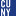CUNY Kingsborough Community College

#### Multiresolution Inverse Wavelet Reconstruction From A Fourier Partial Sum, Nataniel Greene

##### Publications and Research

The Gibbs phenomenon refers to the lack of uniform convergence which occurs in many orthogonal basis approximations to piecewise smooth functions. This lack of uniform convergence manifests itself in spurious oscillations near the points of discontinuity and a low order of convergence away from the discontinuities.In previous work [11,12] we described a numerical procedure for overcoming the Gibbs phenomenon called the Inverse Wavelet Reconstruction method (IWR). The method takes the Fourier coefficients of an oscillatory partial sum and uses them to construct the wavelet coefficients of a non-oscillatory wavelet series. However, we only described the method standard wavelet ...